Courses

# Test: Bar Graphs- 1

## 5 Questions MCQ Test Logical Reasoning (LR) and Data Interpretation (DI) | Test: Bar Graphs- 1

Description
This mock test of Test: Bar Graphs- 1 for UPSC helps you for every UPSC entrance exam. This contains 5 Multiple Choice Questions for UPSC Test: Bar Graphs- 1 (mcq) to study with solutions a complete question bank. The solved questions answers in this Test: Bar Graphs- 1 quiz give you a good mix of easy questions and tough questions. UPSC students definitely take this Test: Bar Graphs- 1 exercise for a better result in the exam. You can find other Test: Bar Graphs- 1 extra questions, long questions & short questions for UPSC on EduRev as well by searching above.
QUESTION: 1

### (Q1-Q5) Directions to solve: The bar graph given below shows the sales of books (in thousand number) from six branches of a publishing company during two consecutive years 2000 and 2001. Sales of Books (in thousand numbers) from Six Branches - B1, B2, B3, B4, B5 and B6 of a publishing Company in 2000 and 2001.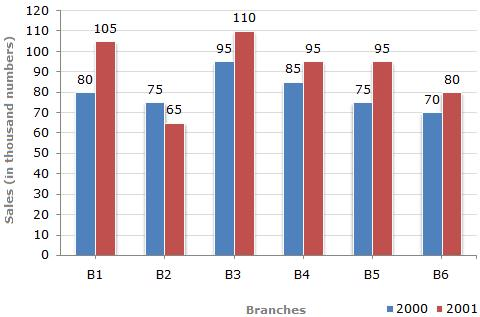Q. What is the ratio of the total sales of branch B2 for both years to the total sales of branch B4 for both years?

Solution:

Required ratio =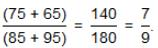QUESTION: 2

### The bar graph given below shows the sales of books (in thousand number) from six branches of a publishing company during two consecutive years 2000 and 2001. Sales of Books (in thousand numbers) from Six Branches - B1, B2, B3, B4, B5 and B6 of a publishing Company in 2000 and 2001.Q. Total sales of branch B6 for both the years is what percent of the total sales of branch B3 for both the years?

Solution:

Required percentage =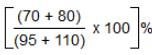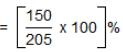= 73.17%

QUESTION: 3

### The bar graph given below shows the sales of books ( in thousand number) from six branches of a publishing company during two consecutive years 2000 and 2001. Sales of Books (in thousand numbers) from Six Branches - B1, B2, B3, B4, B5 and B6 of a publishing Company in  2000 and 2001.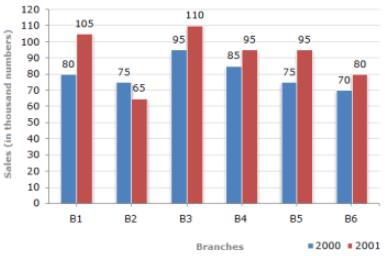Q. What percent of the average sales of branches B1, B2 and B3 in 2001 is the average sales of branches B1, B3 and B6 in 2000?

Solution:

Average sales (in thousand number) of branches B1, B3 and B6 in 2000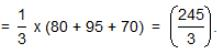Average sales (in thousand number) of branches B1, B2 and B3 in 2001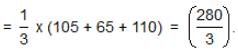∴ Required percentage =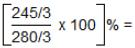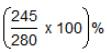= 87.5%

QUESTION: 4

The bar graph given below shows the sales of books (in thousand number) from six branches of  a publishing company during two consecutive years 2000 and 2001.
Sales of Books (in thousand numbers) from Six Branches - B1, B2, B3, B4, B5, and B6 of a publishing Company in 2000 amd 2001.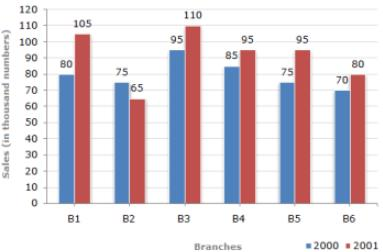Q. What is the average sales of all the branches (in thousand numbers) for the year 2000?

Solution:

Average sales of all the six branches (in thousand number) for the year 2000​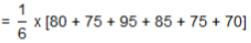= 80.

QUESTION: 5

The bar graph given below shows the sales of books (in thousand number) from six branches of a publishing company during two consecutive years 2000 and 2001.
Sales of Books (in thousand numbers) from Six Branches - B1, B2, B3, B4, B5 and B6 of a publishing Company in 2000 and 2001.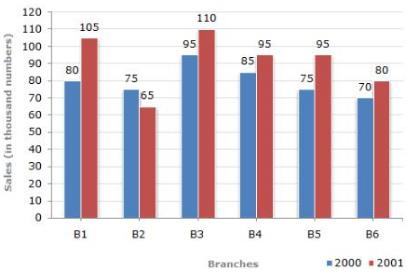Q. Total sales of branches B1, B3 and B5 together for both the years (in thousand numbers) is

Solution:

Total sales of branches B1, B3 and B5 for both the years (in thousand numbers)
= (80 + 105) + (95 + 110) + (75 + 95)
= 560.